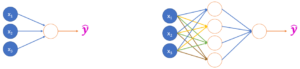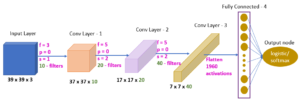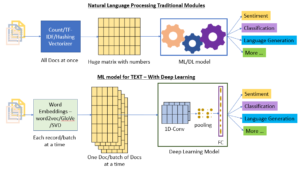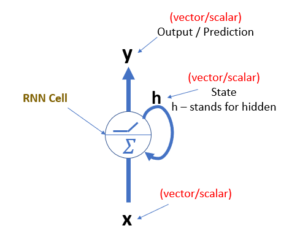What is Deep Learning?

Deep Learning is a branch of Artificial Intelligence, technically an advancement to Machine Learning. In a nutshell, creating a powerful algorithm by combining many homogeneous Machine Learning algorithms is the key.The basis for Deep Learning is Neural Networks. Let’s take a concrete example, in machine learning we use “Logistic Regression” algorithm to train and predict. In deep learning we build “sequence of layers” where each layer contains “many algorithms in parallel”. This makes the model very powerful. In the below image, the shades of green, red circles are machine learning algorithms called as nodes, they will have activation functions such as Logistic Regression, Tanh, Rectified Linear Units (RELU), etc.

The layers with  the “violet nodes” are “input layer / input nodes” which is nothing but a distinct “record in the train data”, the “red node” is the “output layer / target predictor” and all the nodes in between are referred as “hidden layers”. This neural network structure mirrors the way neurons are connected  inside the human brain leading to all the nodes in the hidden layers passing their understanding to final output node. The result will be very powerful model, as all these nodes (algorithms) in hidden and output layer are working cohesively to predict the target value./p>

Machine Learning algorithm will be able to generate decent predictions for moderate number of records and structured/numeric data. Algorithms must be more powerful to bring insights from huge volumes of structured/unstructured data with images, videos, text and numerics. The deep learning is the solution with the following reasons,

• The algorithms have got many neurons to process and understand patterns.
• The algorithms follow stochastic approaches, hence can handle huge volumes/variety and velocity of data, such as big-data.

Types of Neural Networks and their use:

Shallow Neural Networks: The neural network with one or less hidden layers.Deep Neural Networks (DNN): The neural network with more than one hidden layer.Convolutional Neural Networks: The neural networks which use convolutions to extract features out of data are called as Convolutional Neural Networks.

Convolutions: The element wise multiplication (* in python) of two matrices which produces a new matrix. The matrices derived after element wise multiplication are called as        “ Convolution Feature” or “Activation Map” or “Feature Map”.

Convolutional neural networks are best in handing images and videos. The computer vision uses CNN extensively. CNN is better in handling data with huge dimensions, in other words CNN can easily extract best features out of thousands or millions of input features. One dimensional convolutions can be used in extracting features from TEXT also.

Example CNN network:Natural Language Processing and Deep Learning:

Natural Language Processing is an art, science and technology to process text (natural language, the language used by humans). Using NLP, we will extract meaningful numeric features from data. Using Count Vectorization, TF-IDF and Hashing Tokenizer we can extract features. However, when the vocabulary in corpus is huge, these models will create data in gigabytes which practically makes it difficult to train the model.

Deep Learning, especially One-dimensional Convolution Neural Networks can extract features from huge volumes of text data even with tons of vocabulary.

Deep Learning techniques work best with huge data as they follow stochastic approach.Recurrent Neural Networks:

Recurrent Neural Networks are very useful in understanding sequence of activities resulting in a consequence. Real word examples of such things are “sequence of historical Stock Prices affecting next day Price”, “Sequence of words in a sentence affecting probability of next Word”, etc. RNN models are very useful in Natural Language Generation, Speech Recognition, Image Labelling, and more.

RNN Node:RNN Network Example: